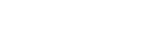# MATH201 - Elements of Mathematics I

## General

MATH

201

00017069

Lecture

### Description

Nature of mathematics from a problem solving approach using sets, relations, number systems, discrete mathematics, and basic algebra. Prereq.: MATH 072 or high school advanced algebra with satisfactory math placement score.

### Notes

Go to www.stcloudstate.edu/mathplacement Placement req prior to Registration

3

3

No

Yes

04

No

## Learning Outcomes

### Outcome

Demonstrate a sound mathematical background, especially in the areas of patterns, relations, functions, discrete mathematics, whole numbers, numeration systems, number theory, integers and rational numbers as fractions, decimals and percentages.

### Outcome

Use problem solving as an integral part of mathematics.

### Outcome

Value and exhibit a positive attitude toward mathematics.

### Outcome

Communicate mathematics clearly and accurately in oral and written form.

### Outcome

Demonstrate an ability to analyze and model mathematical concepts.

### Outcome

Demonstrate an understanding of mathematics and the connections between mathematical concepts themselves as well as connections to other disciplines.

-

## Dependencies

No dependencies720 4th Avenue South
St. Cloud, MN 56301-4498
(320) 308-0121St. Cloud State University
A member of Minnesota State and committed to legal affirmative action, equal opportunity, access and diversity of its campus community (Full Statement).
© 2021 St. Cloud State University